## Goal

In this tutorial we look at some of the data on wealth and life expectancy of countries over time used by Hans Rosling in his famous TED talk. The goal is to introduce some simple 1D and 2D plots constructed using `ggplot2`.

### How to work on this tutorial

• In these notes, I supply some initial plots for a topic, and then pose some questions or extensions as Your turn.

• You will learn best if you try to reproduce them yourself as you go along.

• Open a new R script in R Studio, say, `gapminder-tut.R`.
• Type the lines into the script, and run them as you go.
• If you want to cheat a bit on typing, you can open the gapminder.R script that was created from this in a browser window and use some cut-and-paste.
• There are a lot of steps here, and you may not complete them in this lab session. I urge you to make as much progress as you can today, and treat the rest as homework. Save your script somewhere you can access it later.

### Load the packages we will use here:

``````library(ggplot2)
library(dplyr)    # data munging
library(scales)   # nicer axis scale labels``````

## Data

The data has conviently been packaged in the R package gapminder, a subset of the original data set from (http://gapminder.org). For each of 142 countries, the package provides values for life expectancy, GDP per capita, and population, every five years, from 1952 to 2007.

It is unlikely that you have this package installed, so the first step is to install it and then load it.

``````install.packages("gapminder")
library(gapminder)``````

Tech note: Here is a way to make a script more portable by testing whether a package is available before installing it.

``if(!require(gapminder)) {install.packages("gapminder"); library(gapminder)}``

What variables are available, and what are their names? `str()` is your friend here.

``str(gapminder)``
``````## Classes 'tbl_df', 'tbl' and 'data.frame':    1704 obs. of  6 variables:
##  \$ country  : Factor w/ 142 levels "Afghanistan",..: 1 1 1 1 1 1 1 1 1 1 ...
##  \$ continent: Factor w/ 5 levels "Africa","Americas",..: 3 3 3 3 3 3 3 3 3 3 ...
##  \$ year     : int  1952 1957 1962 1967 1972 1977 1982 1987 1992 1997 ...
##  \$ lifeExp  : num  28.8 30.3 32 34 36.1 ...
##  \$ pop      : int  8425333 9240934 10267083 11537966 13079460 14880372 12881816 13867957 16317921 22227415 ...
##  \$ gdpPercap: num  779 821 853 836 740 ...``````

Tech note: The `gapminder` data set was constructed as a `tibble`, a generalization of a `data.frame`. The `print()` method gives an abbreviated printout. Normally, you would use `head(gapminder)` for this.

``gapminder``
``````## # A tibble: 1,704 x 6
##    country     continent  year lifeExp      pop gdpPercap
##    <fct>       <fct>     <int>   <dbl>    <int>     <dbl>
##  1 Afghanistan Asia       1952    28.8  8425333      779.
##  2 Afghanistan Asia       1957    30.3  9240934      821.
##  3 Afghanistan Asia       1962    32.0 10267083      853.
##  4 Afghanistan Asia       1967    34.0 11537966      836.
##  5 Afghanistan Asia       1972    36.1 13079460      740.
##  6 Afghanistan Asia       1977    38.4 14880372      786.
##  7 Afghanistan Asia       1982    39.9 12881816      978.
##  8 Afghanistan Asia       1987    40.8 13867957      852.
##  9 Afghanistan Asia       1992    41.7 16317921      649.
## 10 Afghanistan Asia       1997    41.8 22227415      635.
## # ... with 1,694 more rows``````

### Overview

Normally, when starting with a new data set, it is useful to get some overview of the variables. The simplest one is `summary()`.

``summary(gapminder)``
``````##         country        continent        year         lifeExp           pop              gdpPercap
##  Afghanistan:  12   Africa  :624   Min.   :1952   Min.   :23.60   Min.   :6.001e+04   Min.   :   241.2
##  Albania    :  12   Americas:300   1st Qu.:1966   1st Qu.:48.20   1st Qu.:2.794e+06   1st Qu.:  1202.1
##  Algeria    :  12   Asia    :396   Median :1980   Median :60.71   Median :7.024e+06   Median :  3531.8
##  Angola     :  12   Europe  :360   Mean   :1980   Mean   :59.47   Mean   :2.960e+07   Mean   :  7215.3
##  Argentina  :  12   Oceania : 24   3rd Qu.:1993   3rd Qu.:70.85   3rd Qu.:1.959e+07   3rd Qu.:  9325.5
##  Australia  :  12                  Max.   :2007   Max.   :82.60   Max.   :1.319e+09   Max.   :113523.1
##  (Other)    :1632``````

We will want to look at trends over time by `continent`. How many countries are in this data set in each continent? There are 12 years for each country. Are the data complete? `table()` gives an answer.

``table(gapminder\$continent, gapminder\$year)``
``````##
##            1952 1957 1962 1967 1972 1977 1982 1987 1992 1997 2002 2007
##   Africa     52   52   52   52   52   52   52   52   52   52   52   52
##   Americas   25   25   25   25   25   25   25   25   25   25   25   25
##   Asia       33   33   33   33   33   33   33   33   33   33   33   33
##   Europe     30   30   30   30   30   30   30   30   30   30   30   30
##   Oceania     2    2    2    2    2    2    2    2    2    2    2    2``````

Tech note: `table()` doesn’t have a `data=` argument, so you have to qualify the names of variables using `data\$variable` notation. Another way to do this is to use the `with()` function, that makes variables in a data set available directly. The same table can be obtained using:

``with(gapminder, {table(continent, year)})``

## 1D plots: Bar plots for discrete variables

Bar plots are often used to visualize the distribution of a discrete variable, like `continent`. With `ggplot2`, this is relatively easy:

• map the `x` variable to `continent`
• add a `geom_bar()` layer, that counts the observations in each category and plots them as bar lengths.
``ggplot(gapminder, aes(x=continent)) + geom_bar()``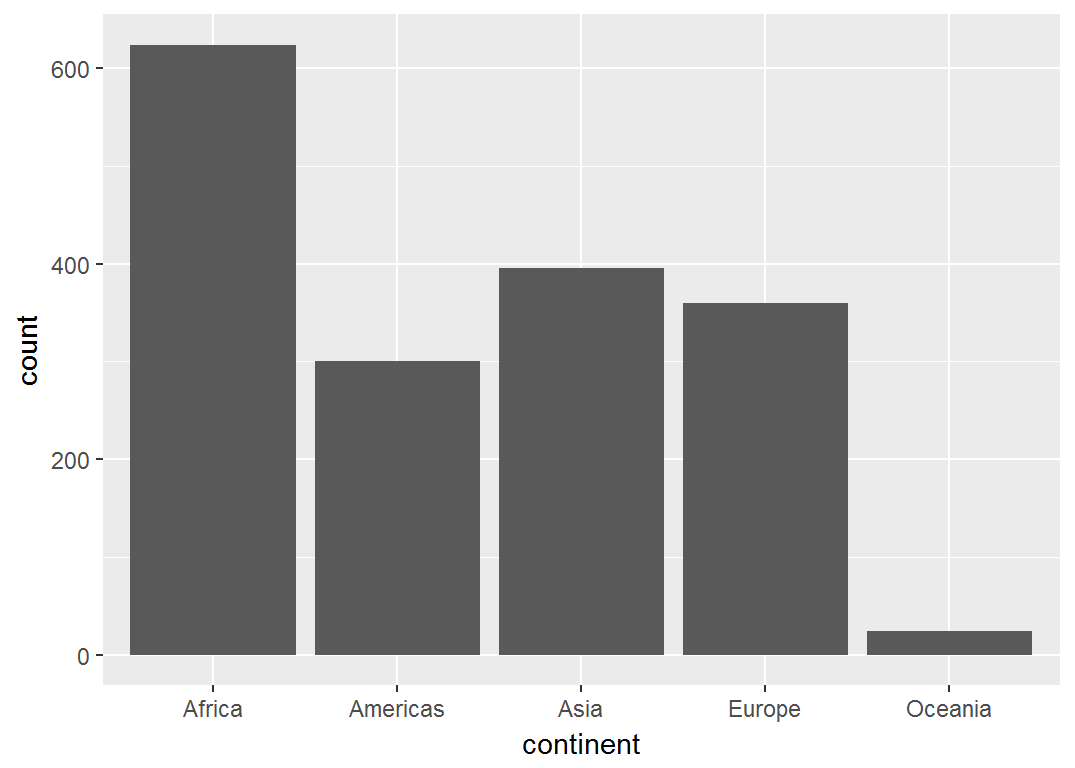To make this (and other plots) more colorful, you can also map the `fill` attribute to `continent`. Note that adding the `fill` attribute automatically adds a legend.

``ggplot(gapminder, aes(x=continent, fill=continent)) + geom_bar()``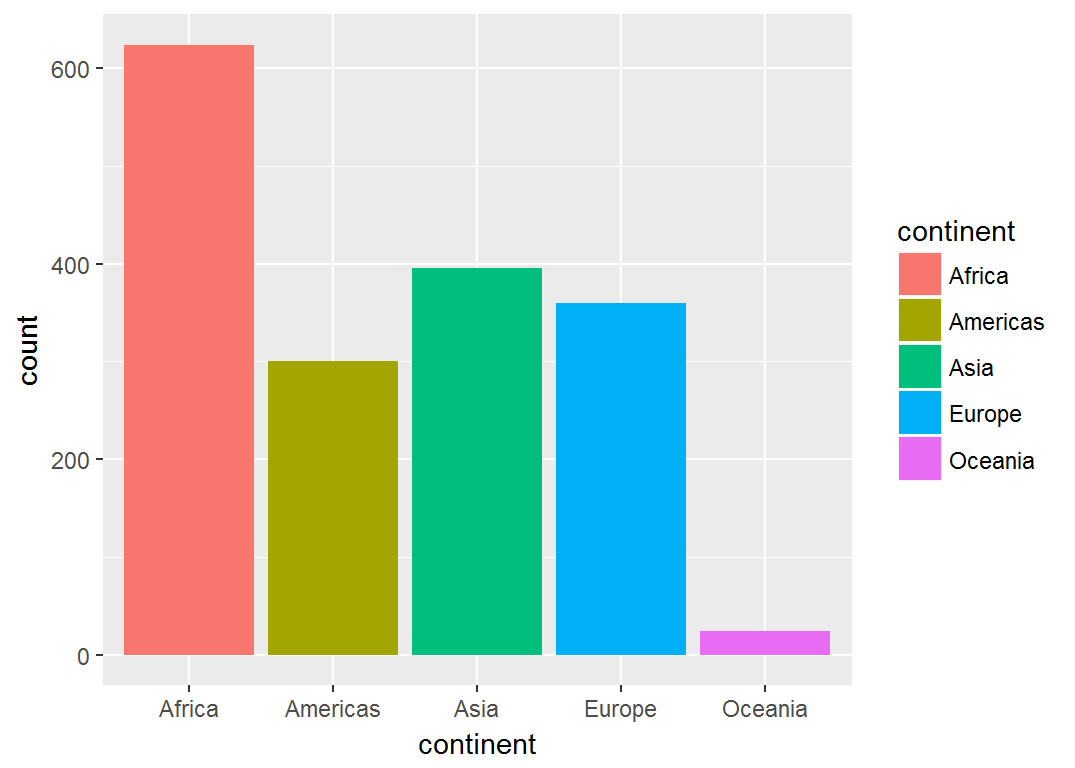As you progress with `ggplot2`, you will also learn to:

• change the default color schemes
• modify labels: text, font, font size, etc.
• change the legend position, or eliminate it when it is not needed
• plot a transformation of a variable
• flip (x, y) coordinates to (y,x)

The next step shows several of these features:

• in `geom_bar` we transform the computed `..count..` to `..count../12` so it represents the number of countries
• add a better label for the `y` axis
• suppress the default legend for `continent`, which is redundant here, because the continents are labeled on the x axis.
``````ggplot(gapminder, aes(x=continent, fill=continent)) +
geom_bar(aes(y=..count../12)) +
labs(y="Number of countries") +
guides(fill=FALSE)``````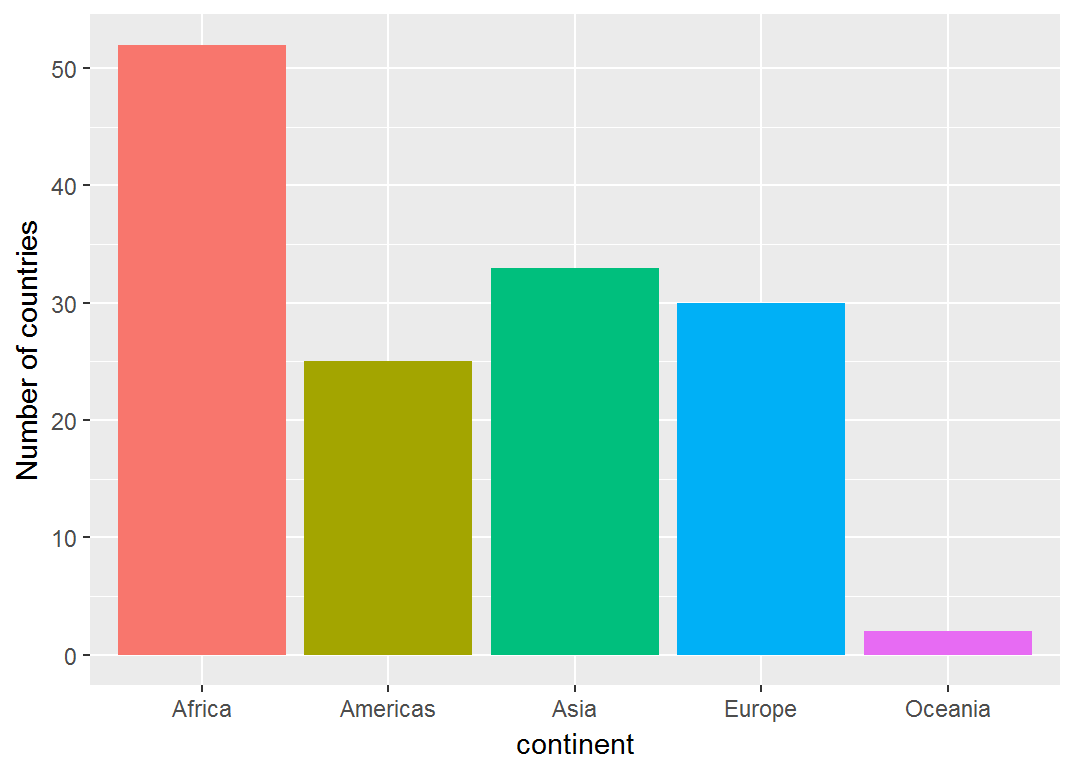Another `ggplot2` feature is that every plot is a `ggplot` object. If you like a given plot, you can save it to some name by using `mybar <- ggplot() + ...`, or after you’ve done it, using `mybar <- last_plot()`

``````# record a plot for future use
mybar <- last_plot()``````

Then, we can add other layers or transform the coordinates with `coord_` functions.

• `coord_trans(y="sqrt")` plots the frequencies on a square root scale
• `coord_flip()` interchanges the X, Y axes, to make a horizontal bar chart.
• `coord_polar()` maps (X,Y) to polar coordinates (radius, angle); a coxcomb chart rather than a pie chart.
``mybar + coord_trans(y="sqrt")``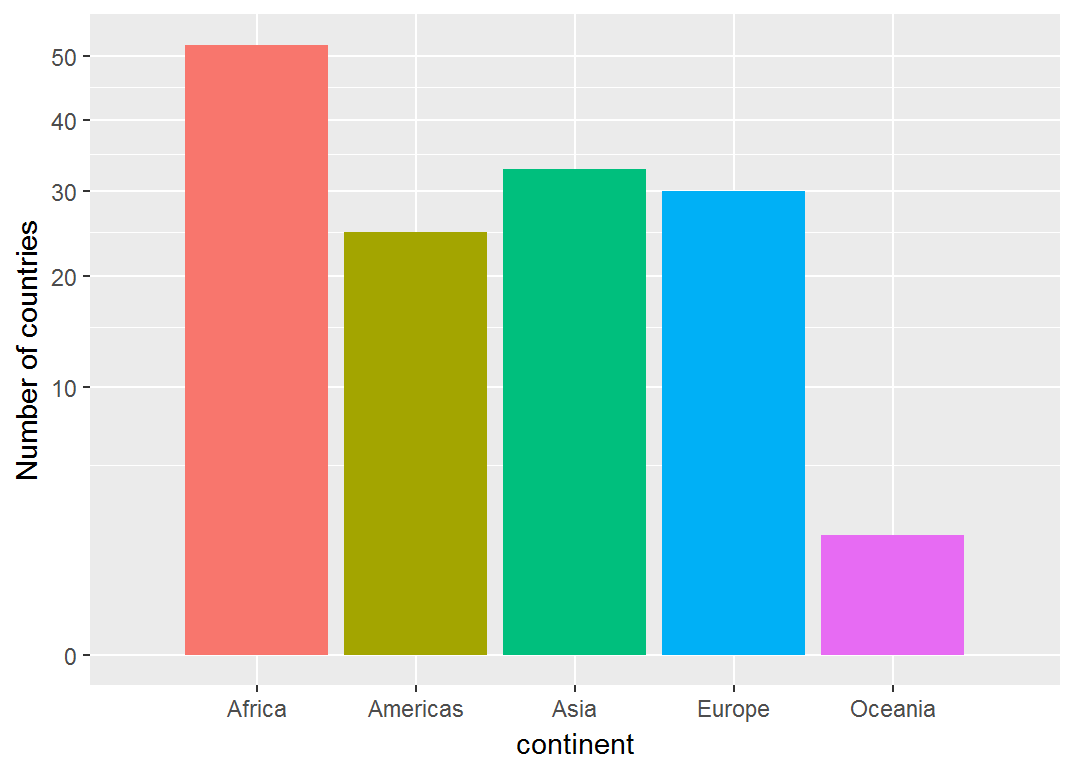``mybar + coord_flip()``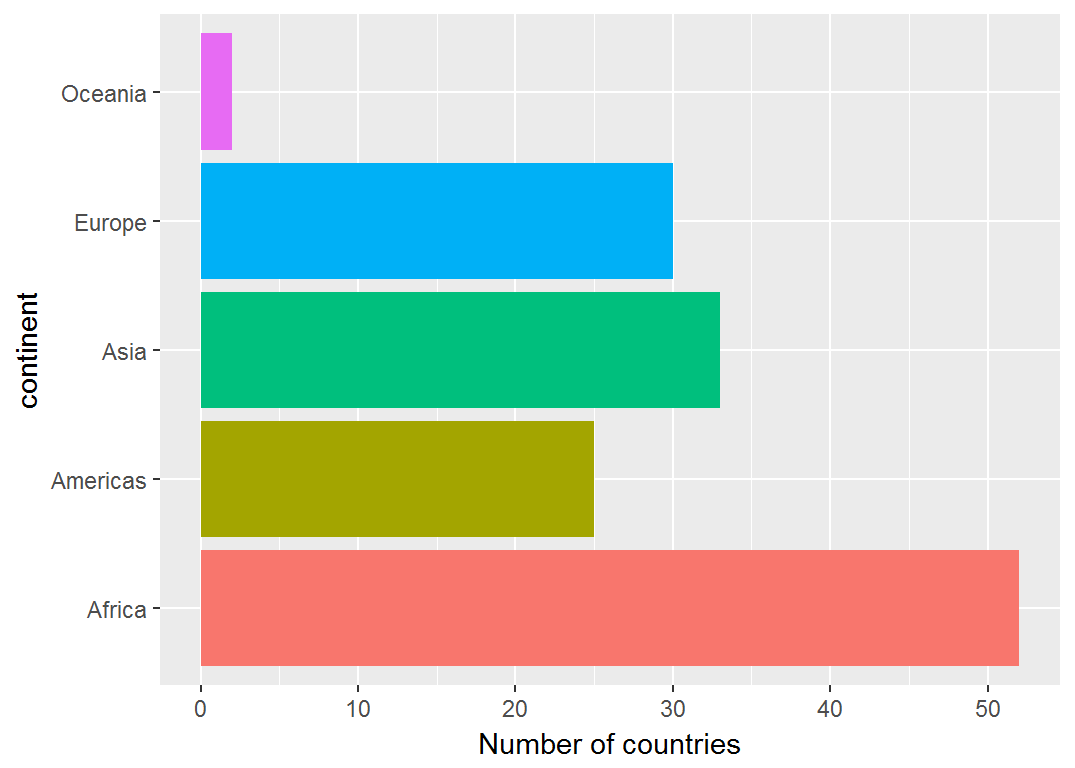``mybar + coord_polar()``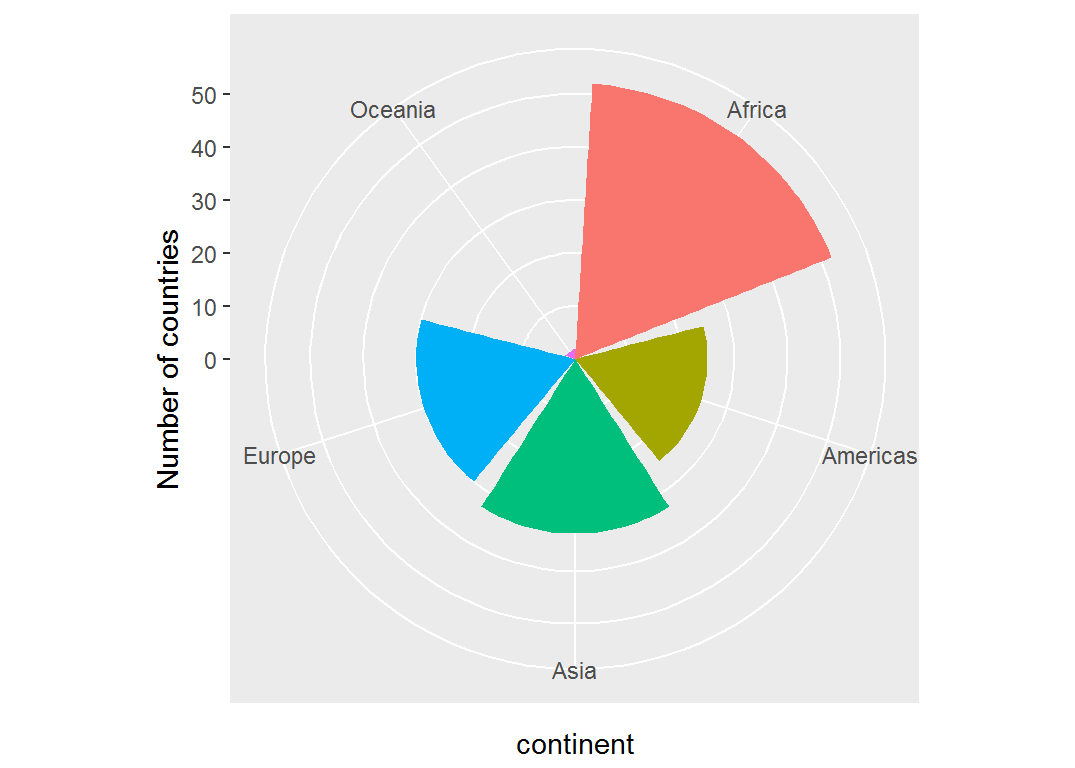## 1D plots: density plots for continuous variables

There are several continuous variables in this data set: life expectancy (`lifeExp`), population (`pop`) and gross domestic product per capita (`gdpPercap`) for each `year` and `country`. For such variables, density plots provide a useful graphical summary.

We will start with `lifeExp`. The simplest plot uses this as the horizontal axis, `aes(x=lifeExp)` and then adds `geom_density()` to calculate and plot the smoothed frequency distribution.

``````ggplot(data=gapminder, aes(x=lifeExp)) +
geom_density()``````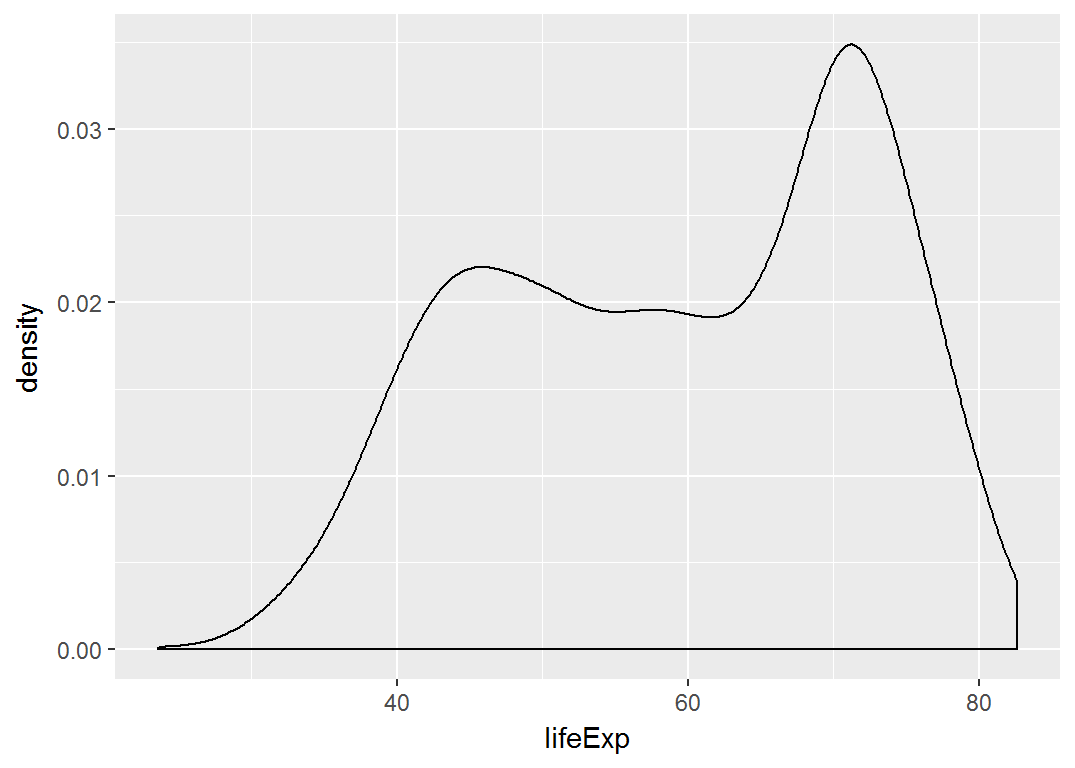To make such plots prettier, you can change the line thickness (`size=`), add a fill color (`fill=""`), and make the fill color partially transparent (`alpha=`). You can see the grid lines underneath the following plots

``````ggplot(data=gapminder, aes(x=lifeExp)) +
geom_density(size=1.5, fill="pink", alpha=0.3)``````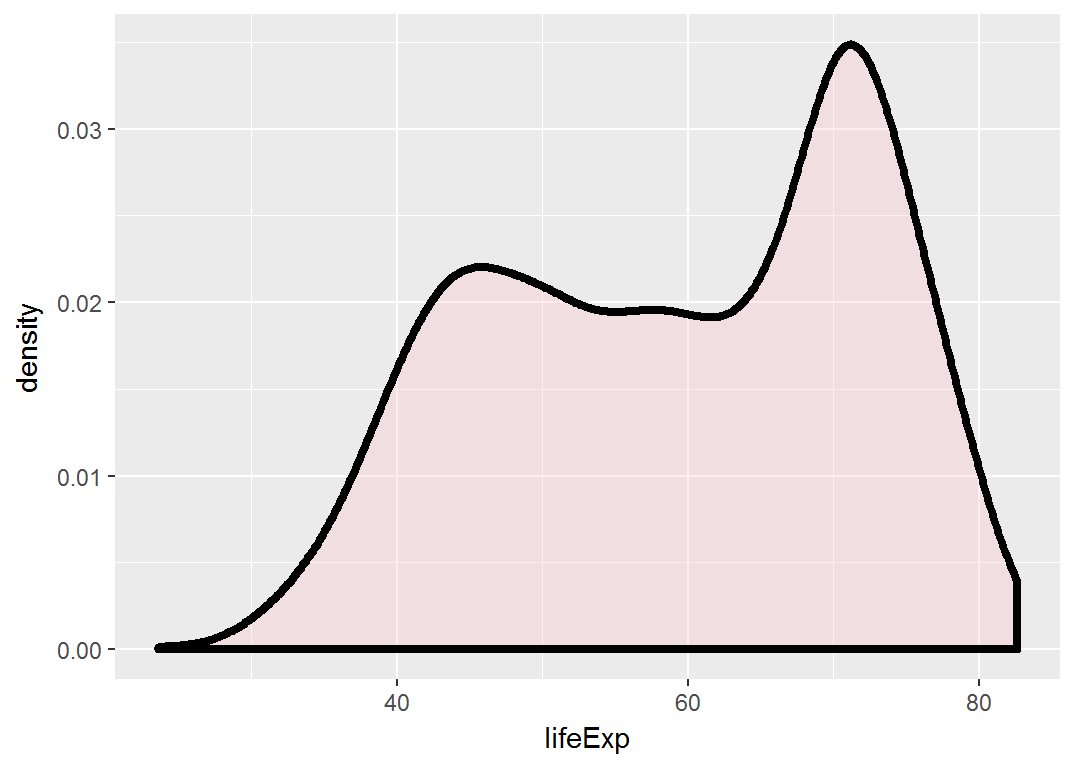If you want, you can also add a histogram later. This is a little more complicated to get right, because historams are computed differently and need some additional arguments.

``````ggplot(data=gapminder, aes(x=lifeExp)) +
geom_density(size=1.5, fill="pink", alpha=0.5) +
geom_histogram(aes(y=..density..), binwidth=4, color="black", fill="lightblue", alpha=0.5)``````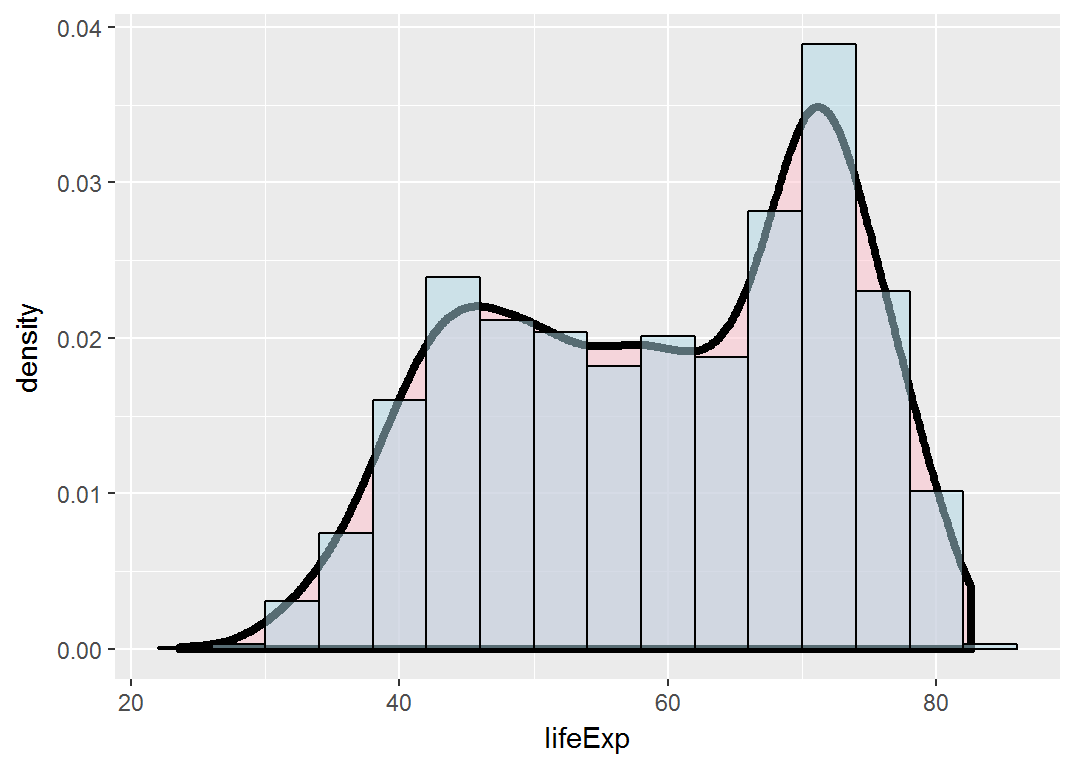I don’t recommend such plots, because the density plot usually gives most of the information, and the histogram doesn’t add much.

### Differences by continent

The plot of `lifeExp` is bimodal, and looks wierd. Maybe this is hiding a difference among countries in different continents. The easy way to see this in `ggplot2` is to add another aesthetic attribute, `fill=continent`, which is inherited in `geom_density()`. Using transparent colors (`alpha=`) makes it easier to see the different distributions across `continent`.

``````ggplot(data=gapminder, aes(x=lifeExp, fill=continent)) +
geom_density(alpha=0.3)``````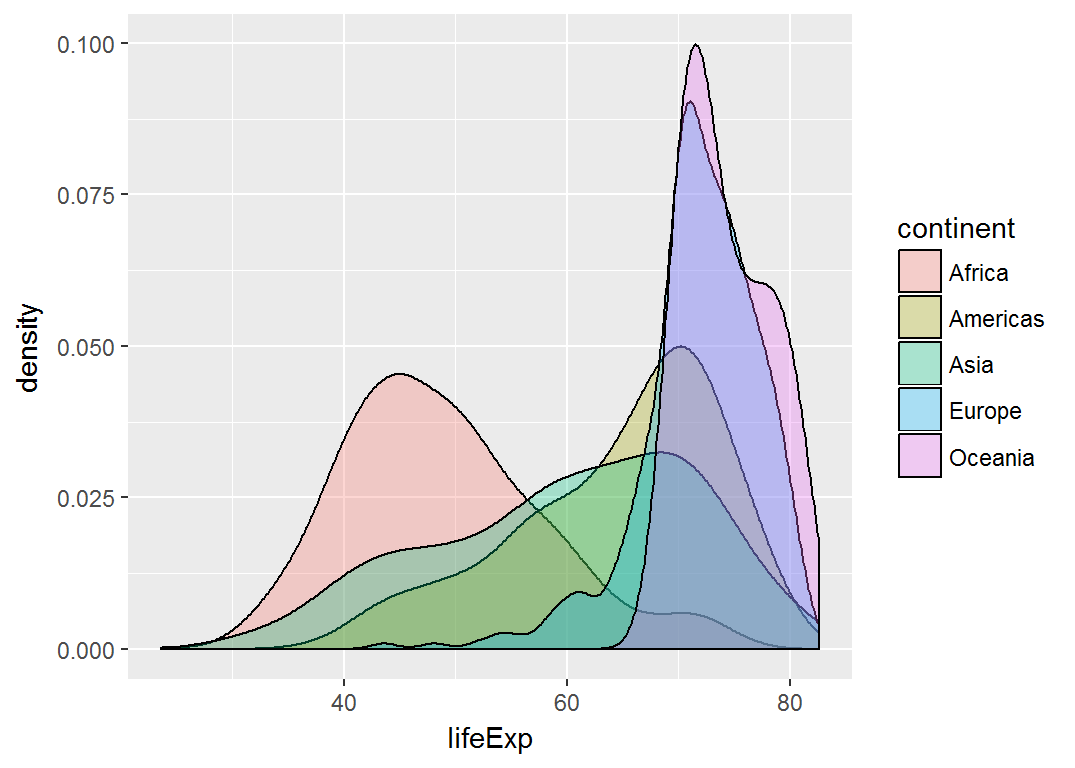It is easy to see that African countries differ markedly from the rest.

### boxplots and other visual summaries

Alternatively, you might want to view the distributions of life expectancy by another visual summary, grouped by `continent`. All you need to do is change the aesthetic to show `continent` on one axis, and life expectancy (`lifeExp`) on the other.

``gap1 <- ggplot(data=gapminder, aes(x=continent, y=lifeExp, fill=continent))``

Then, add a `geom_boxplot()` layer:

``````gap1 +
geom_boxplot(outlier.size=2)``````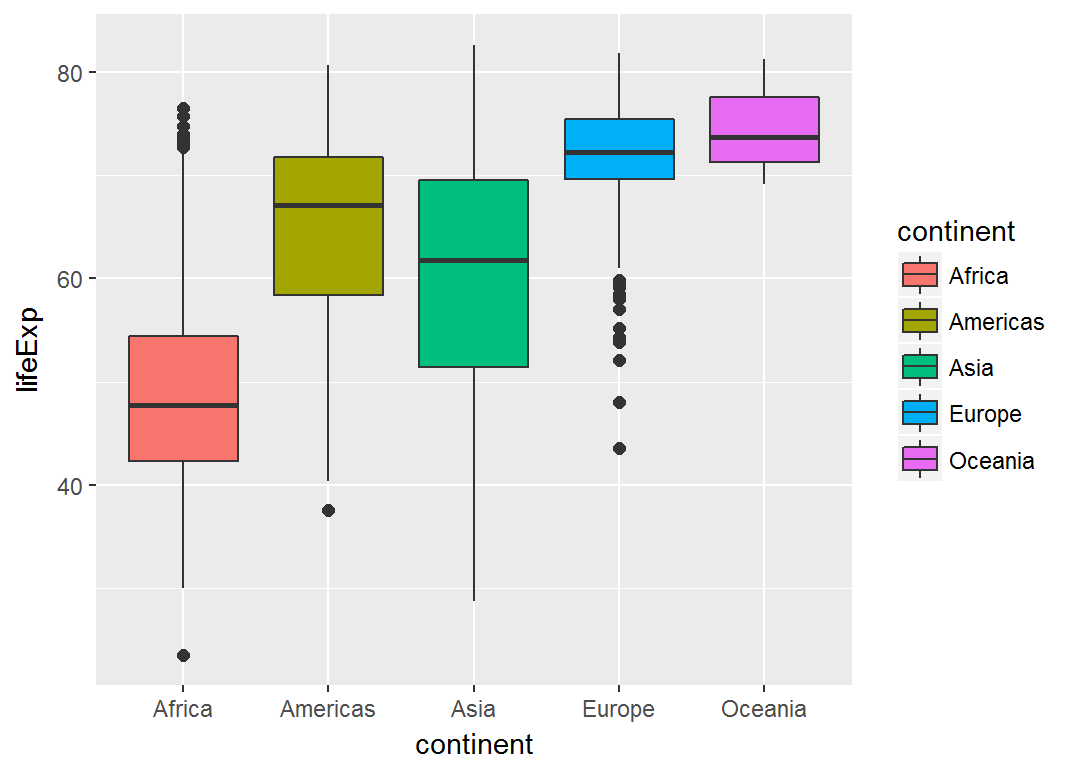• Remove the legend from this plot
• Also, make the plot horizontal
• Instead of a boxplot, try `geom_violin()`

### Effect ordering

The continents are a factor, and are ordered alphabetically by default. It might be more useful to order them by the mean or median life expectancy.

In this example, I use the `dplyr` “pipe” notation (`%>%`) to send the `gapminder` data to the `dplyr::mutate()` function, and within that, `reorder()` the continents by their median life expectancy.

``````gapminder %>%
mutate(continent = reorder(continent, lifeExp, FUN=median))``````

(In other situations, you could use `FUN=mean`, `FUN=sd`, or `FUN=max` to sort the levels by their means, standard deviatons, maximums, or any other function.)

Continuing from here, you can pipe the result of this right into `ggplot`:

``````gapminder %>%
mutate(continent = reorder(continent, lifeExp, FUN=median)) %>%
ggplot(aes(x=continent, y=lifeExp, fill=continent)) +
geom_boxplot(outlier.size=2)``````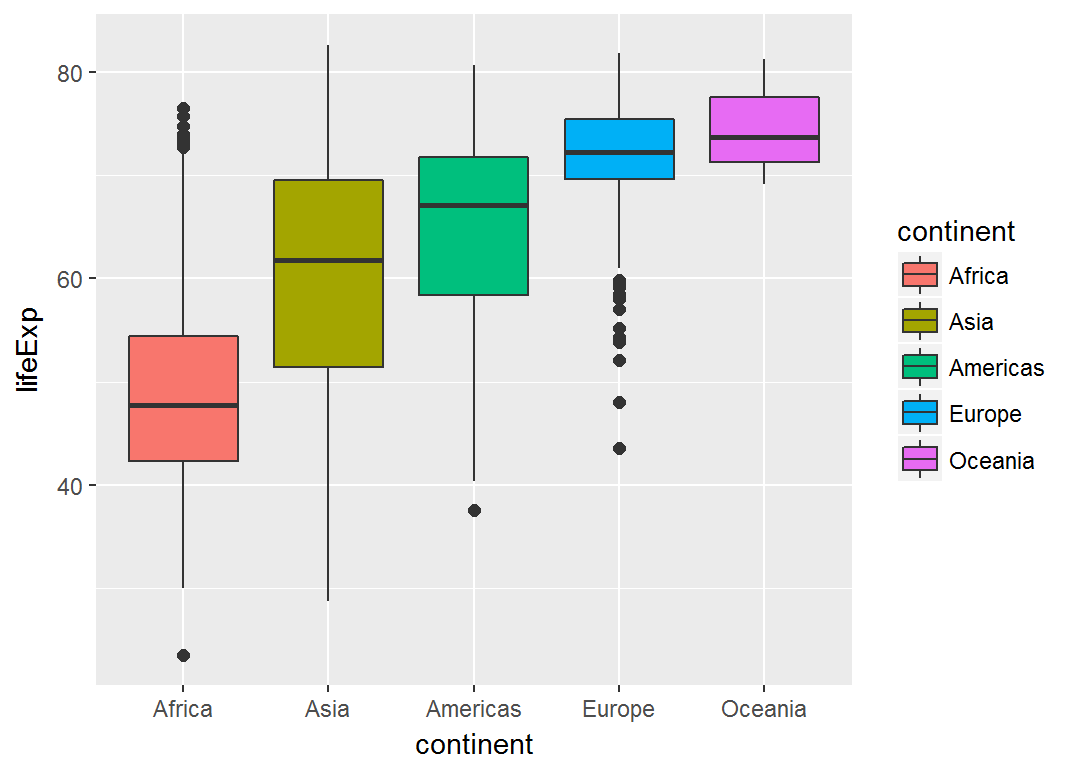### Looking at GDP

Let’s look at the distribution of `gdpPercap` in a similar way, starting with the unconditional distribution.

``````ggplot(data=gapminder, aes(x=gdpPercap)) +
geom_density()  ``````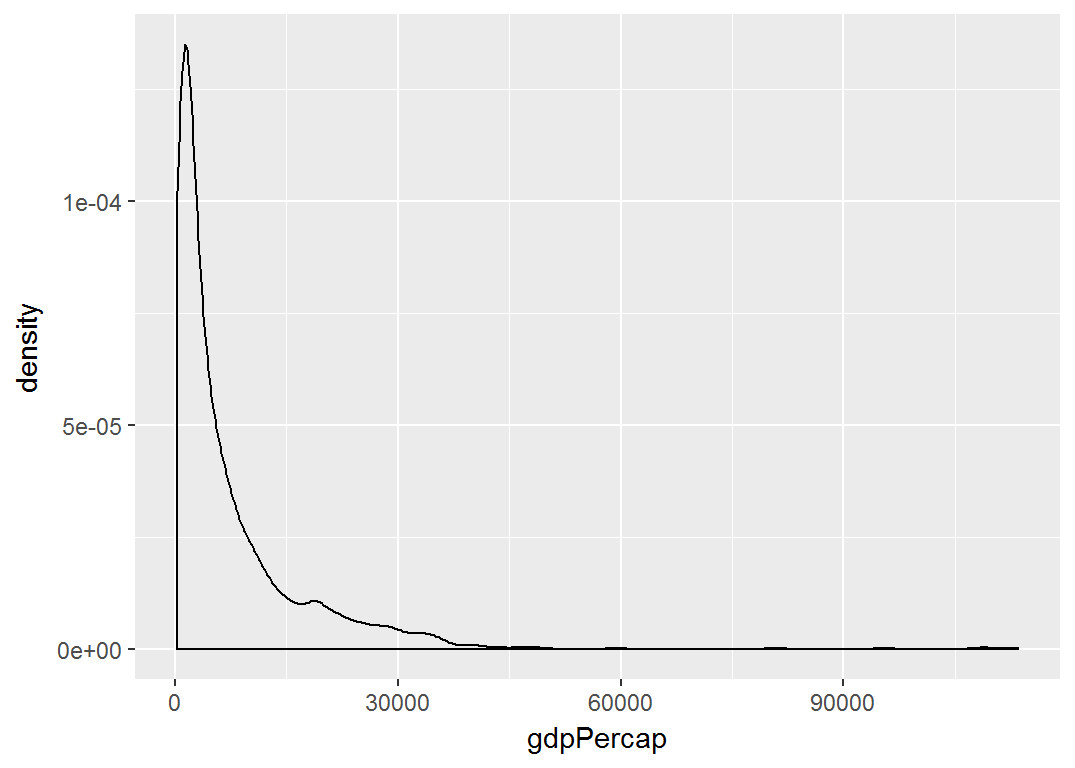Hmm, that is extremely positively skewed.

• As we did for `lifeExp` plot the distributions separately for each continent
• It is probably more useful to plot GDP on a log scale. Add another layer that transforms the `x` axis to `log10(gdpPercap)`.
• Make boxplots of `gdpPercap` by `continent`
How has life expectancy changed over time? The simplest way to to plot a line for each country over `year`. To do this, we use the `group` aesthetic.
``````ggplot(gapminder, aes(x=year, y=lifeExp, group=country)) +